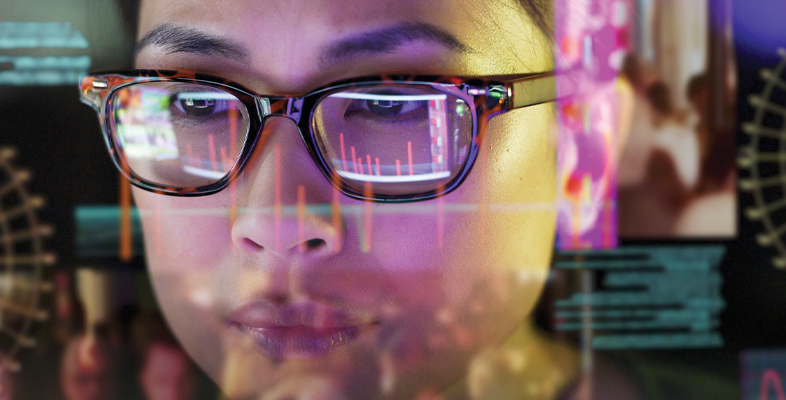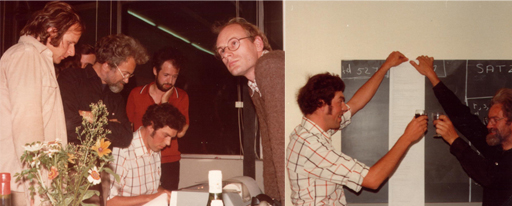Digital thinking tools for better decision making

Start this free course now. Just create an account and sign in. Enrol and complete the course for a free statement of participation or digital badge if available.

Free course

# 2.2 Computers as tools for mathematicians

Working mathematicians continued their labours and were relatively unfazed by Gödel’s and Turing’s findings. If anything, they were reassured that computers wouldn’t put them out of a job anytime soon.

With the rise of computing technology, some forays were made into turning computers into the mathematician’s assistant or tool. Whereas there is no general computer program for telling whether mathematical statements are true, there are relatively simple programs that can check whether a proof of a statement is correct. In other words, although computers can’t come up with a proof for any arbitrary statement, they can tell us whether a proof, once found, has no missing or incorrect steps.

An early example is the Automath project by Dick de Bruijn (1918–2012) and his research group at Eindhoven University of Technology in the Netherlands. In the 1960s, the Automath team checked the proofs in a classic textbook on the foundations of analysis (Landau’s Grundlagen der Analysis) – see Figure 9. Several years of labour went into translating the textbook content into precise statements that could be processed by a computer. Along the way several mistakes and gaps in the original ‘proofs’ were discovered and corrected.Figure 9 The Automath team using a computer to check the final proof of Landau’s mathematics textbook, followed by celebrations with two team members holding a print-out of the final proof.

Automath pioneered the idea that computers can play a useful role in assisting, rather than replacing, mathematicians. The need for such tools has become increasingly pressing as some of the most influential mathematical proofs can take over a hundred pages of dense mathematical reasoning.

A dramatic example is the famous proof by Andrew Wiles (born 1953) of Fermat’s last theorem. The initial proof of well over a hundred pages contained a mistake that was discovered only after Wiles had announced his proof in public. Wiles succeeded in correcting the proof and no further issues have been reported since.

Some mathematicians have warmed to using proof checkers to verify mathematical results, despite the enormous amount of work that is involved in translating a human-authored proof to computer input. For instance, after six years, in 2012, a team from Inria (the French national research institute for the digital sciences) and Microsoft Research completed checking a significant mathematical theorem, the Feit–Thompson theorem (Breton, 2012).

DTT_1